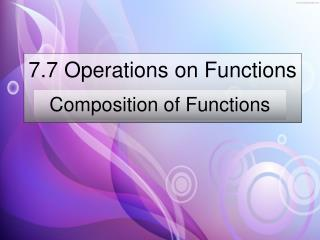DownloadDownload Presentation7.7 Operations on Functions

# 7.7 Operations on Functions

Download Presentation## 7.7 Operations on Functions

- - - - - - - - - - - - - - - - - - - - - - - - - - - E N D - - - - - - - - - - - - - - - - - - - - - - - - - - -
##### Presentation Transcript

1. 7.7 Operations on Functions Composition of Functions

2. A new way of writing Operations with functions Adding Subtracting Multiplication Division

3. Given Find

4. Given Find

5. Given Find

6. Given Find

7. Given Find

8. Given Find

9. Given Find

10. Given Find

11. Given Find

12. Composition of Functions Combining two functions into one function. Where the answers to one function is the input to the other function. Given two Functions. f(x) = 2x + 1 g(x) = x – 3 f(g(x))=2(x – 3)+1 f(g(x))=2x – 6+1= 2x - 5 Where ever there is an x, put the other function

13. Composition of Functions Given two Functions. f(x) = 2x + 1 g(x) = x – 3 f(g(x))=2(x – 3)+1 f(g(x))=2x – 6+1= 2x – 5 g(f(x))=(2x + 1) – 3 = 2x - 2

14. Composition of Functions“The Books Notation” Given two Functions. f(x) = 2x + 1 g(x) = x – 3 f(g(x))=2(x – 3)+1 [f○ g](x) f(g(x))=2x – 6+1= 2x – 5 [f○ g](x)= 2x - 5 g(f(x))=(2x + 1) – 3 = 2x - 2 [g ○ f ](x) = 2x - 2

15. Find [ f ○ g](x) and [ g ○ f ](x) for x = -2 f(x) = 3x2 – x + 4; g(x) = 2x – 1 [ f ○ g](x) find g(-2) = 2(-2) – 1 = -5 find f(-5)

16. Find [ f ○ g](x) and [ g ○ f ](x) for x = -2 f(x) = 3x2 – x + 4; g(x) = 2x – 1 [ f ○ g](x) find g(-2) = 2(-2) – 1 = -5 find f(-5) = 3(-5)2 – (-5) + 4 =3(25) + 5 + 4 =75 + 5 +4 = 84

17. Find [ f ○ g](x) and [ g ○ f ](x) for x = -2 f(x) = 3x2 – x + 4; g(x) = 2x – 1 [ g ○ f](x) find f(-2) = 3(-2)2 – (-2) + 4 =3(4) + 2 + 4 =12 + 2 + 4 = 18 find g(18) =

18. Find [ f ○ g](x) and [ g ○ f ](x) for x = -2 f(x) = 3x2 – x + 4; g(x) = 2x – 1 [ g ○ f](x) find f(-2) = 3(-2)2 – (-2) + 4 =3(4) + 2 + 4 =12 + 2 + 4 = 18 find g(18) = 2(18) - 1 =36 - 1 =35

19. Homework Page 387 – 388 # 17, 20, 31, 32, 35 – 43 odd

20. Homework Page 387 – 388 # 18, 21, 33, 36 – 44 even